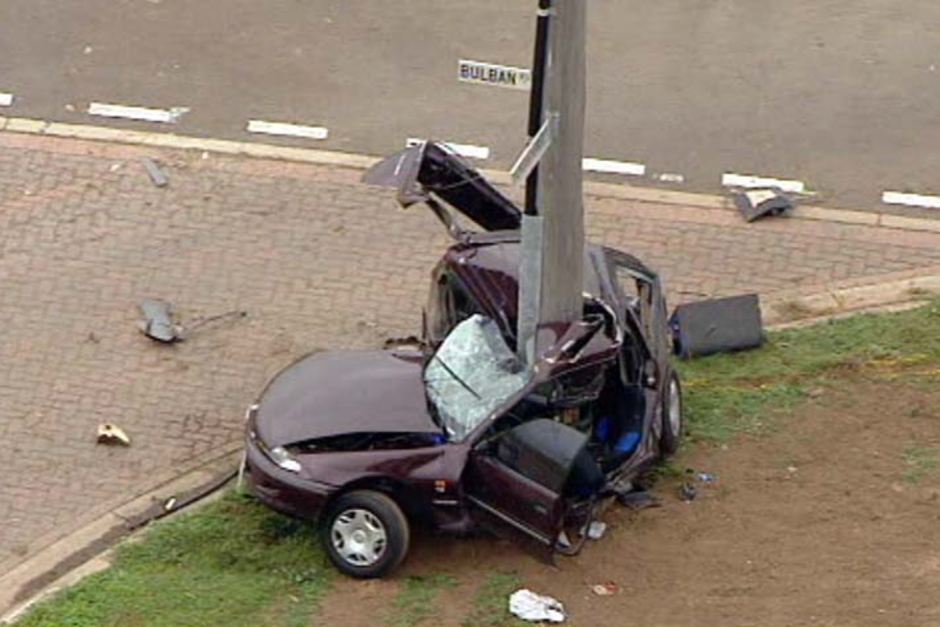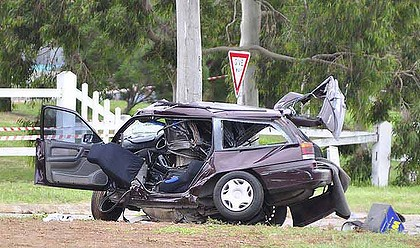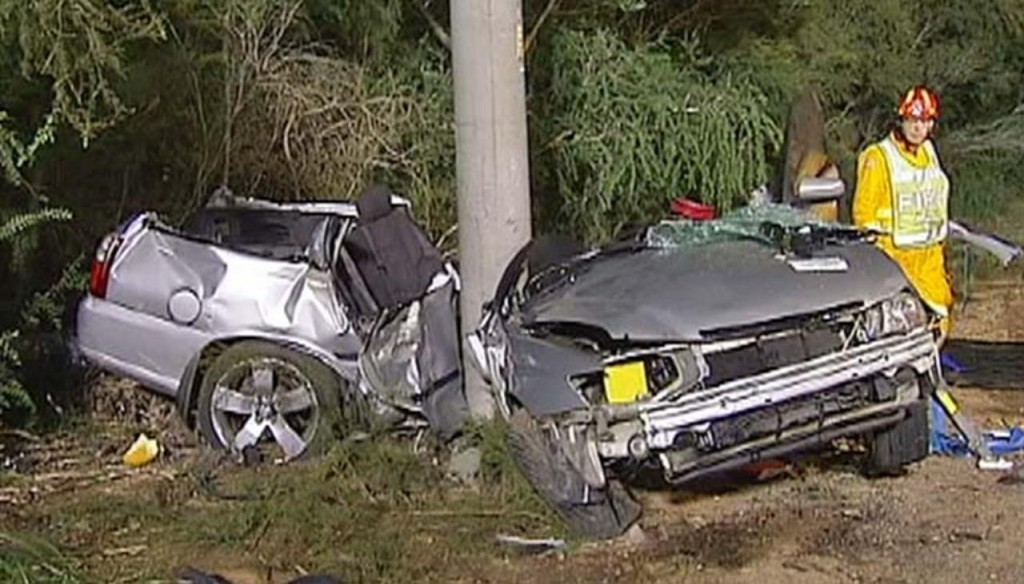# Kinetic energy – when a vehicle stops suddenly, the energy has to go somewhere

Posted by: Gary Ernest Davis on: March 26, 2011It’s an intuitive feeling that when we are driving a car, doubling our speed doubles our danger.

Sadly, this intuition is not correct.

Doubling our speed quadruples our danger.

The momentum of a car is the product of its weight$m$ (mass) and its speed$v$ (strictly speaking, the product of its mass and velocity).

Momentum doubles if the speed of a car doubles.

However, the kinetic energy – called the “living energy” by Leibniz – or the energy of motion, is$\frac{1}{2}mv^2$.

That speed squared term in the kinetic energyÂ  means that if the speed increases from$v$ to$2v$ – doubles in other words – the kinetic energy goes from$\frac{1}{2}mv^2$ to$\frac{1}{2}m(2v)^2= 4\times \frac{1}{2}mv^2$.

That is, doubling a car’s speed leads to a quadrupling of its kinetic energy.

Because energy is conserved, if a car stops suddenly the kinetic energy – the energy of motion – has to go somewhere.A lot of that energy will be transferred to energy of motion of the passengers – usually throwing them from the car and killing or seriously injuring them.

That square term in kinetic energy is one strong reason why speed can kill.